# KCSE 2017 Mathematics Alt A Paper 2 with Marking Scheme

INSTRUCTIONS TO CANDIDATES

• This paper consists of two sections: Section I and Section II
• Answer all questions in Section I and only five questions from Section II.
• Marks may be given for correct working even if the answer is wrong
• Non-programmable silent electronic calculator and KNEC Mathematical tables may be used, except where stated otherwise
• Candidates should answer the questions in English

SECTION I (50 marks)

Answer all the questions in this section in the spaces provided.

1. The roots of a quadratic equation are x = −3/5  and x = 1. Form the quadratic equation in the form ax2 +bx+c = 0 where a, b and care integers.   (2 marks)
2. A tailor intended to subdivide a piece of cloth into 7 equal parts. She approximated 1/7m to 0.14 m. Calculate the percentage error in the approximation  (3 marks)
3. A miller was contracted to make porridge flour to support a feeding program. He mixed millet, sorghum, maize and Omena in the ration 1:2:5:1. The cost per kilogram of millet was Ksh 90. sorghum Ksh 120, maize Ksh 30 and omena Ksh 150.
Calculate:
1. the cost of one kilogram of the mixture;  (2 marks)
2. the selling price of 1 kg of the mixture if the miller made a 30% profit.   (2 marks)
4. Without using mathematical tables or a calculator, evaluate 5/6 log1064 + log1050 - 4log102.  (3 marks)
5. In the figure below, PQRS is a cyclic quadrilateral. PQ- QR,  ∠PQR =105° and PS is parallel to QR.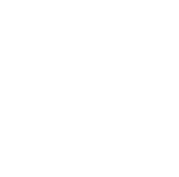Determine the size of:
1. ∠PSR;   (1 mark)
2. ∠PQS.   (2 marks)
6. Make t the subject of
s=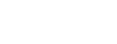(3 marks)
7. Simplify      3
3 − √7      (2 marks)
8. Using a ruler and a pair of compasses only, construct:
1. a triangle LMN in which LM=5 cm, LN = 5.6 cm and Z MLN = 45°    (2 marks)
2. the circle that touches all the sides of the triangle    ( 2marks)
9. The figure below is a triangle ABC.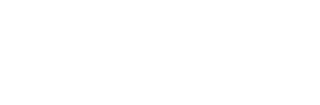1. On the triangle, locate the focus of points equidistant from AC and AB and 5 cm from B.  (2 marks)
2. Shade the region R, inside the triangle, which is less than 5 cm from B and nearer to AC than AB  (1 mark)
10. An aircraft took off from a point P (65° S, 76° W) and few due North to a point Q. The distance between P and Q is
5400 nm. Determine the position of Q.   (3 marks)
11. The equation of a circle is x2 + y2 - 4x + 6y + 4 = 0. On the grid provided, draw the circle.   (4 marks)
12. Determine the amplitude, period and the phase angle of the curve: (3 marks)
y = 5/2 sin(4θ +60°)
13. A basketball team scored the following points in 6 matches: 10, 12, 14, 16, 28 and 30. Using an assumed mean of 15. determine the standard deviation correct to 2 decimal places.   (4 marks)
14. A committee of 3 people was chosen at random from a group of 5 men and 6 women. Find the probability that the committee consisted of more men than women.   (4 marks)
15. The area of a triangle is 24 square units. The triangle is mapped onto image P by the matrix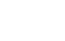Find the area of the image P. 3 4       (2 marks)
16. Given that OA = 3i + 4j + 7k, OB = 4i + 3j +9k and OC = i +6j + 3k,  show that points A, B and C are collinear.
(3 marks)

SECTION II (50 marks)

Answer any five questions from this section in the spaces provided.

1. The income tax rates of a certain year were as shown in the table below:
 Monthly taxable income in Kenya shillings(Ksh) Tax rate percentage(%) in each shilling 0 to 9680 10 9681 to 18 800 15 18801 to 27920 20 27921 to 37040 25 37041 and above 30
In that year, Shaka's monthly earnings were as follows:
Basic salary                            Ksh 28600
House allowance                     Ksh  15000
Medical allowance                   Ksh  3200
Transport allowance                 Ksh  540
Shaka was entitled to a monthly tax relief of Ksh 1056.
1. Calculate the tax charged on Shaka's monthly earnings.   (6 marks)
2. Apart from income tax, the following monthly deductions were made; a Health Insurance fund of Ksh 500, Education Insurance of Ksh 1 200 and 2% of his basic salary for widow and children pension scheme. Calculate Shaka's monthly net income from his employment.   (4 marks)
2. The vertices of a rectangle ABCD are: A(0,2), B(0,4), C(4,4) and D(4,2). The vertices of its image under a transformation T are;A'(0,2), B'(0,4), C'(8,4) and D'(8,2).
1.
1. On the grid provided, draw the rectangle ABCD and its image A'B'C'D' under T (2 marks)
2. Describe fully the transformation T.    (3 marks)
3. Determine the matrix of transformation.    (2 marks)
2. On the same grid as in (a), draw the image of rectangle ABCD under a shear with line x=-2 invariant and A(0, 2) is mapped onto A"(0,0).               (3 marks)
3.
1. The table below shows values of x and some values of y for the curve y = x3 − 2x2 − 9x + 8 for −3 ≤ x ≤ 5. Complete the table.
 x −3 −2 −1 0 1 2 3 4 5 y = x3 − 2x2 − 9x + 8 −10 14 8 −10 4
(2 marks)
2. On the grid provided, draw the graph of y = x3 − 2x2 − 9x + 8 for −3 ≤ x ≤ 5
Use the scale; 1 cm represents 1 unit on the x-axis
2 cm represents 10 units on the y-axis        (3 marks)
3.
1. Use the graph to solve the equation y = x3 − 2x2 − 9x + 8 = 0.   (1 mark)
2. By drawing a suitable straight line on the graph, solve the equation
x3 − 2x2 − 11x + 6 = 0       (4 marks)
4. The figure below represents a cuboid ABCDEFGH in which AB = 16 cm, BC = 12 cm and CF = 6 cm.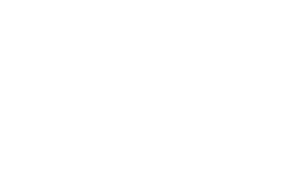1. Name the projection of the line BE on the plane ABCD.  (1 mark)
2. Calculate, correct to I decimal place:
1. the size of the angle between AD and BF;   (2 marks)
2. the angle between line BE and the plane ABCD;   (3 marks)
3. the angle between planes HBCE and BCFG.   (2 marks)
3. Point N is the midpoint of EF. Calculate the length BN, correct to 1 decimal place.   (2 marks)
5. Three quantities X, Y and Z are such that X varies directly as the square root of Y and inversely as the fourth root of Z. When X = 64, Y = 16 and Z = 625.
1. Determine the equation connecting X, Y and Z.  (4 marks)
2. Find the value of Z when Y = 36 and X = 160.   (2 marks)
3. Find the percentage change in X when Y is increased by 44%  (4 marks)
6. A trader stocks two brands of rice A and B. The rice is packed in packets of the same size. The trader intends to order fresh supplies but his store can accommodate a maximum of 500 packets. He orders at least twice as many packets of A as of B. He requires at least 50 packets of B and more than 250 packets of A. If he orders x packets of A and y packets of B,
1. Write the inequalities in terms of x and y which satisfy the above information. (4 marks)
2. On the grid provided, represent the inequalities in part a) above.  (4 marks)
3. The trader makes a profit of Ksh 12 on a packet of type A and Ksh 8 on a packet of type B rice. Determine the maximum mprofit the trader can make.  (2 marks)
7.
1. The 5th term of an AP is 82 and the 12th term is 103.
Find:
1. the first term and the common difference;   (3 marks)
2. the sum of the first 21 terms.   (2 marks)
2. A staircase was built such that each subsequent stair has a uniform difference in height. The height of the 6th stair from the horizontal floor was 85 cm and the height of the 10th stair was 145 cm.
Calculate the height of the 1st stair and the uniform difference in height of the stairs.    (3 marks)
3. During the construction of the staircase, each step was supported by a vertical piece of timber. If the staircase has 11 stairs, calculate the total length of timber used.   (2 marks)
8. The length of a room is 3 m shorter than three times its width. The height of the room is a quarter of its length. The area of the floor is 60 m2.
1. Calculate the dimensions of the room.   (5 marks)
2. The floor of the room was tiled leaving a border of width y m, all round. If the area of the border was 1.69 m2, find:
1. the width of the border,   (4 marks)
2. the dimensions of the floor area covered by tiles.   (1 mark)## MARKING SCHEME

1. (x − 1)(5x + 3) = 0
5x2 − 5x + 3x − 3 = 0
5x2 − 2x − 3 = 0
Accept (x − 1)(x + 3/5) = 0
2. lErrorl = 1/7 − 14/100
= 1/350
% Error = 1/350 ÷ 1/7 × 100
= 1/350 × 7/1 × 100
= 2%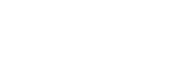3.
1. M:S:M:O = 1:2:5:1
Cost of 1 kg of mixture
= 90 + 2(120) + 5(30) + 150
9
=630
9
=Kah 70
2. 130/100 × 70
Ksh 91
4.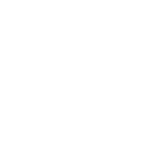= 2
5.
1. ∠ PSR = 180 − 105 = 75°
2. ∠ PQS = ∠SRP
∠ SRP = 180 − (37.5 + 75)
= 67.5°
6. S2 = 3d (t − d)
8
8S2 = 3dt − 3d2
t = 8S2 + 3d2
3d

7.     3      × 3 + √7
3 − √7     3 + √7
=3(3 + √7)
9−7
= 9 + 3√
8.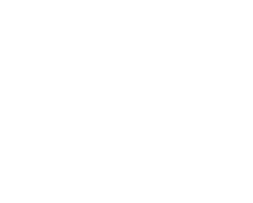9.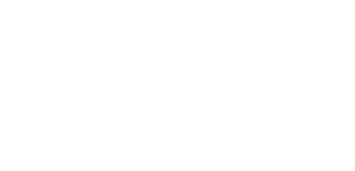10. d = 60 × θ
5400 = 60 × θ
5400 = θ
60
θ = 90°
Position of Q is (25°N, 76°W)
ALT
(65 − x) 60 = 5400
x = −25
Q(25°N, 70°W)
11. x2 − 4x + (−2)2 + y2 + 6y + (3)2 = −4 + (−2)2 + (3)2
(x − 2)2 + (y+3)2 = 32
Centre (2, −3), r = 3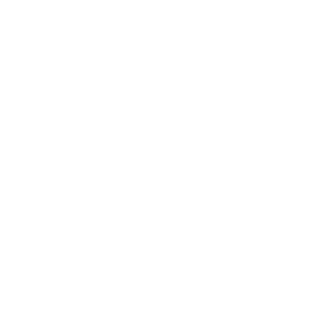12. y = 5/2 sin (4θ + 60°)
Amplitude = 2½
Period = 90°
Phase angle = 60°
13.  Score d = x − a d2 10    12    14    16    28    30 −5     −3     −1      1     13     15 25    9    1    1   169      225 n = 6 Σd = 20 Σd2 = 430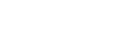= √60.56
= 7.78
14.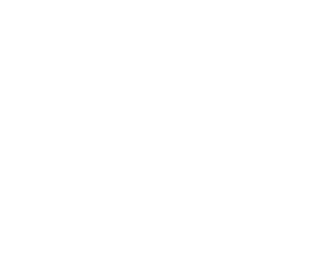P(more men than women)
P(more men than women)
=P(MMW or MWM or WMM)
=(5/11 × 4/10 × 6/9) + (5/11 × 6/10 × 4/9) + (6/11 × 5/10 × 4/9)
4/334/334/33
12/33 or 4/11
15. det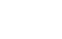= 24 − 15 = 9
Area of image = 9 × 42
= 216sq units
16.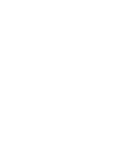AC = −2AB
AB//AC
and A is a commoon point
A, B and C are collinear
17.
1. Total earning/ Taxable income
= Ksh (28600 + 15000 + 3200 + 540)
= Ksh 47340
Total charged:
Up to 9680 → 9680 × 10% = Ksh 968
9681 - 18800 → 9120 × 15%  = Ksh 1368
18801 - 27920 → 9120 × 20% = Ksh 1824
27924 - 37040 →  9120 × 25% = Ksh 2280
Above 37040 → 10300 × 30% = Ksh 3090
Total tax less relief:
(968 + 1368 + 1824 + 2280 + 3090) − 1056
= Ksh 8474
2. Monthly deductions:
2% of Ksh 28600 = Ksh 572
Total deductions
Ksh (8474 + 500 + 1200 + 572)
= Ksh 10746
Ney income = Ksh (47340 − 10746)
= Ksh 36594
18.
1.

1.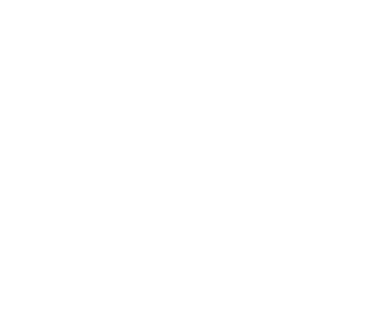ABCD correctly drawn and labelled
A'B'C'D' correctly drawn and labelled
2. T is a stretch
S. F. = 2
x=0 or y- axis variant
3. T =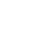2. Invariant line identified and used
A'', B'', C'', and D'' plotted
A'', B'', C'', and D'' drawn correctly
19.
1.
 x −3 −2 −1 0 1 2 3 4 5 y = x3 − 2x2 − 9x + 8 −10 10 14 8 −2 −10 −10 4 38
2.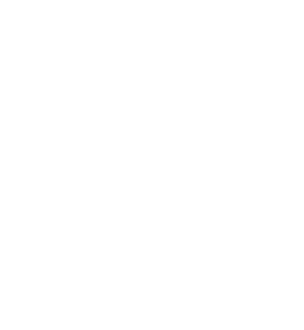3.
1. Roots x = −2.6, 0.8, 3.8 Or for (0,0), x = −2.6, 0, 3.8
2. y = x3 − 2x2 − 9x + 8
0 = x3 − 2x2 − 11x + 6
y =                   2x + 2
y = 2x + 2 drawn
Roots are − 2.7, 0.5, 4.3
For (0,0); x = − 2.7, −0.1 and 4.3
20.
1. Projection of BE is BD
2.
1. Angle between line AD and BF
=tan−1 (6/12)
=26.6°
2. Angle between line BE and plane ABCD
BD = √(122 + 162)
= 20
Tan (DBE) = 6/20
∠ DBE = tan−1 (6/20)
= 16.7°
3. Angle between HBCE abd BCFG
= tan−1 (16/6)
=69.4°
3. BF = √(122 + 62)
= √180
BN =√(180 + 82)
= 15.6cm
21.
1.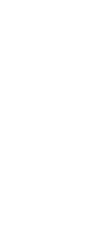2.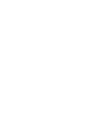3.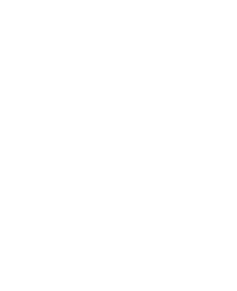22.
1. x + y ≤ 500
x ≥ 2y
y ≥ 50
x > 250
2.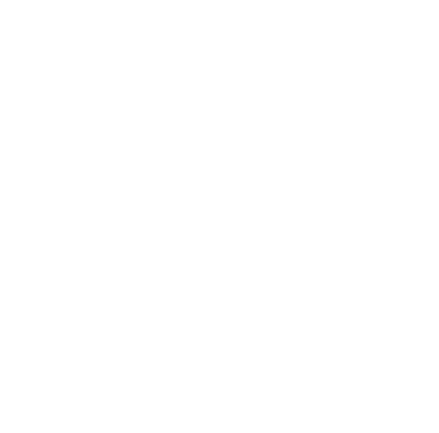x + y ≤ 500
x ≥ 2y
y ≥ 50
x > 250
3. Search line 12x + 8y = 4000
For maximum profit x = 450, y = 50
Maximum profit = 12 × 450 + 50 × 8
= ksh 5800
23.
1.
1. an = a + (n − 1)d
a5 = a + 4d = 82
a12 = a + 11d = 103
7d = 21
d = 3
a + 4(3) = 82
a = 70
2. Snn/2(2a + (n − 1)d
S2121/2(2(70)+ 20(3)
=2100
2. a + 5d = 85
a + 9d =145
4d = 60
d = 15cm
a + 5(15) = 85
a = 10cm
3. Snn/2(2a + (n − 1)d)
S1111/2 (2(10) + 10(15))
=935cm
24.
1. Let x be the width
(3x − 3)x = 60
3x2 − 3x − 60 = 0
x2 − x − 20 = 0
(x − 5)(x + 4) = 0
x = 5 or x = −4
∴ width x = 5m
Length = 12m
Height = 3m
2.
1. 60 − (12−2y)(5−2y) = 1.69
34y − 4y2 = 1.69
4y2 − 34y + 1.69 = 0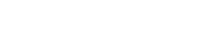y = 8.45 or y = 0.05
∴ y = 0.05m
2. Dimensions or tiled area
Length = 12 − 0.1 = 11.9m
Width = 5 − 0.1 = 4.9m

#### Download KCSE 2017 Mathematics Alt A Paper 2 with Marking Scheme.

• ✔ To read offline at any time.
• ✔ To Print at your convenience
• ✔ Share Easily with Friends / Students